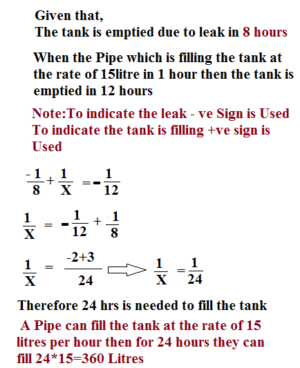# LET US SOLVE PROBLEMS ON PIPES & CISTERN-HOW TO SERIES

Hi Bankersdaily Aspirants,

How is your exam preparation going on?I think all were in the hot seat & busy in the preparation most of us would curiously ask our friends about the technique he used to solve the problem.Friends if you are alone and you don’t have that person X(Friend) to torture & ask for the Technique then consider us your friend and feel free to ask your doubt .If you were not aware of any topic in Aptitude & Reasoning,If you were new to this Banking Career then take a look on Learn Series were we had discussed on each topic from Basic

### INTRODUCTION:

In this Article we are going to look some of the problems from Pipes & Cistern.Friends before looking into the problem let us look into Basic in Pipes & Cistern

1)A leak can empty a tank in 8 hours.A Pipe which fills the tank at the rate of 15 litres per hour and due to this the tank is emptied after 12 hours.Then find the Capacity of the tank?

First I will explain by Partial Fraction Method that most of the Aspirants choose to solve the Problem.

EXPLANATIONNow let us solve the same sum using our Method Shall we Start

Aspirants Keep your Paper & Pen Aside Let us Solve without using it.Answer my Question alone

### What is given in the question?

The tank can be emptied in 8 Hours due to leak

If the tank is filled at the rate of 15 litres per hour then it is emptied in 12hours

### LCM of 8,12=24Litre

Now Let us Calculate the Efficiency

Tank empties in 8 Hours:

Efficiency=24/8 =3 litre/hour

When Tank Empties in 12 Hours due to filling :

Efficiency=24/12=2 litre/hour

### Now What is the Difference between the Efficiency(3-2=1)

So,time taken=Total Capacity/Efficiency⇒24/1=24hour

### Original Capacity of the tank:

Given that the Pipe fill the tank at the rate of 15 litres per hour

=24*!5

=360Litres

Therefore the Capacity of the tank=360 Litre.

Friends from our Method we can solve the problem by just taking LCM of the given number & Finding the difference between their efficiency,

2)Two Pipes can fill the tank in the tank in 26 hours and 39 hours respectively.Due to leakage in the tank the tank is filled after 2 hour & 24 minutes.Find how long will the leak take to empty the tank,if the tank is full,What is the efficiency of the leak?

EXPLANATION

Given that,

Two Pipes can fill the tank in 26 hours & 39 hours respectively,

I think now you came to practice that if we don’t know the value we would take LCM of the given number

LCM(26,39)=468

Therefore the Total Capacity of the tank=468 Litres

Efficiency of 1st Pipe=468/26=18 litres/hour

Efficiency of 2nd Pipe=468/39=12 litres/hour

Total Efficiency when two Pipes are opened=12+18=30 litres/hour

Time Taken to fill the tank=468/30=15 hours 36 minutes

Given that it takes extra 2 hours & 24 Minutes to fill the tank due to leak in the tank.

Therefore the Time taken=15 hours 36 Minutes + 2 hours 24 Minutes

Total Time Taken=18 hours

When Efficiency=468/18=26 litres/hour.

From this it is understood that When 2 Pipes are opened they can fill 30 litres/hour & Due to leak they can fill 26 litres per hour Which indicates the Leak drains 4 litres/hour

So,Time taken to empty the fill tank=468/4=117 hours

3)Two Pipes can fill the Tank in 15 minutes and 30 minutes respectively.There is an outlet Pipe which is in the middle of the tank & it has the capacity of draining 30 litres per minute.If all the Pipes are open then the tank can be filled in 25 minutes.What is the capacity of the tank?

EXPLANATION

Given that the Tank is filled by 2 Inlet Pipes in just 15 minutes & 30 minutes And there is one Outlet Pipe in the middle of the tank & When all the pipes are opened together then the tank is filled in 25 minutes

Here we don’t know the Capacity of the Tank,So as always we should take LCM of the given numbers

Capacity of the tank=LCM(15,30,25)

Capacity of the tank=150litres

To Find Efficiency,

Efficiency=Total Capacity/Time Taken

Efficiency of 1st Pipe=150/15=10 Litres/minute

Efficiency of 2nd Pipe=150/30=5 Litres/Minute

It is given that the Outlet Pipe is in the middle of the Tank

Therefore

150/2=75litres of the tank is filled by 2 inlet pipe without wasting any water

Therefore Time taken to fill the tank When both the Inlet Pipes are Open=75/15=5 Minutes

It is given that When all the Pipes are opened together then the tank is filled in 25 minutes

So half of the tank (75 Litres) is filled in 5 minutes

Remaining Time=25 Minutes-5 Minutes=20 Minutes

Efficiency of three Pipe=Remaining Capacity/Time

=75/20=15/4Litres/Minute

To know the Time taken by the Outlet Pipe:

Efficiency of 2 Inlet Pipe-Efficiency of all the pipe(

=15 – 15/4

=45/4Litre/Minute

Time taken by the Outlet Pipe=Capacity/Efficiency

=150*4/45

=40/3 Minute

Given that Outlet Pipe can Pump out 30 litres in 1 Minutes therefore in 40/3 Minute it can Pump=400 litres

Therefore the Capacity=400 Litres

Friends don’t just panic by seeing the Length of the Question.I had solved all the problems intentionally in LCM Method in order to show that any difficult problem can be solved by LCM Method and By Just knowing What we have learned in the Basic Class and by Just knowing the Relationship Between the 3 Terms